# Profit and Loss Questions for Class 7

Profit and Loss Questions for Class 7, deals with various concepts which are as under:-

• Find the Gain when Cost Price and Selling Price is Given
• Find the Loss when Cost Price and Selling Price is Given
• Find the Gain % when Cost Price and Selling Price is Given
• Finding Loss % when Cost Price and Selling Price is given
• Find Gain and Gain %
• Find Loss and Loss%
• Find Cost Price when Selling Price & Loss Percent is given
• Find Cost Price when Selling Price & Gain Percent is given
• Find Selling Price when Cost Price & Loss Percent is given
• Find Selling Price when Cost Price & Gain Percent is given

#### Profit and Loss Questions for Class 7 – Find the Gain when Cost Price and Selling Price is Given

Question 1

If Cost Price = ₹ 10 and Selling Price = ₹ 15 , then find the gain?

Explanation:

Cost Price (CP) – Price at which an Article is purchased.

Selling Price (SP) – Price at which the Article is sold.

Gain – Where the Selling Price is greater than the Cost Price, the difference between the SP and CP is the gain

In the present case,

CP = ₹ 10

SP = ₹ 15

We know that,

Gain = SP – CP

Gain = ₹ ( 15 – 10 )

Gain = ₹ 5

Hence, Gain = ₹ 5

#### Profit and Loss Questions for Class 7 – Find the Loss when Cost Price and Selling Price is Given

Question 2

If Cost Price = ₹ 30 and Selling Price = ₹ 25 ; Find Loss?

Explanation:

Cost price(CP) – Price at which an article is purchased.

Selling price(SP) – Price at which an article is sold.

Loss – Where the Cost Price is greater than the Selling Price, the difference between the SP and CP is the Loss

In present case,

CP = ₹ 30

SP = ₹ 25

We know that,

Loss = CP – SP

Loss = ₹ ( 30 – 25 )

Loss = ₹ 5

#### Profit and Loss Questions for Class 7 – Find the Gain % when Cost Price and Selling Price is Given

Question 3

If Cost Price = ₹ 1200 and Selling Price = ₹ 1500 , Find gain percent?

Explanation:

Cost Price (CP) – Price at which an Article is purchased.

Selling Price (SP) – Price at which the Article is sold.

Gain – Where the Selling Price is greater than the Cost Price, the difference between the SP and CP is the gain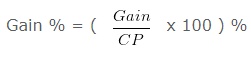In the present case,

CP = ₹ 1200

SP = ₹ 1500

We know that,

Gain = SP – CP

Gain = ₹ ( 1500 – 1200 ) = ₹ 300

Further,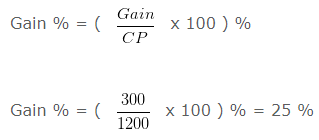Hence, Gain % = 25 %

#### Profit and Loss Questions for Class 7 – Finding Loss % when Cost Price and Selling Price is given

Question 4

If Cost Price = ₹ 90 and Selling Price = ₹ 72 , Find Loss percent?

Explanation:

Cost price(CP) – Price at which an article is purchased.

Selling price(SP) – Price at which an article is sold.

Loss – Where the Cost Price is greater than the Selling Price, the difference between the SP and CP is the Loss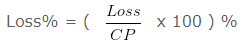In present case,

CP = ₹ 90

SP = ₹ 72

We know that,

Loss = CP – SP

Loss = ₹ ( 90 – 72 )

Loss = ₹ 18

Further, we also know that,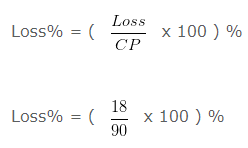Loss% = 20 %

Hence, Loss% = 20 %

#### Profit and Loss Questions for Class 7 – Find Gain and Gain %

Question 5

An Article was bought for ₹ 1250 and sold for ₹ 1500 .Find the Gain and Gain%?

Explanation:

CP of an Article = ₹ 1250

SP of an Article = ₹ 1500

Since, SP > CP

So, there is Gain

We know that,

Gain = SP – CP

Gain = ₹ 1500 – ₹ 1250

Gain = ₹ 250

Further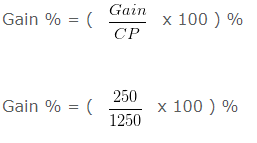Gain % = 20 %

Hence, Gain = ₹ 250 and Gain % = 20 %

#### Profit and Loss Questions for Class 7 – Find Loss and Loss%

Question 6

An Article was bought for ₹ 500 and sold for ₹ 450. Find Loss or Loss%?

Explanation:

CP of an Article = ₹ 500

SP of an Article = ₹ 450

Since, SP < CP

So, there is Loss

We know that,

Loss = CP – SP

Loss = ₹ 500 – ₹ 450

Loss = ₹ 50

Further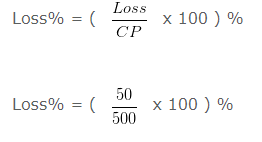Loss% = 10 %

Hence, Loss = ₹ 50 and Loss% = 10 %

#### Profit and Loss Questions for Class 7 – Find Cost Price when Selling Price & Loss Percent is given

Question 7

Find the Cost Price of an Article whose Selling Price = ₹ 3200 and Loss% = 60 % ?

Explanation:

Cost price(CP) – Price at which an article is purchased.

Selling price(SP) – Price at which an article is sold.

Loss – Where the Cost Price is greater than the Selling Price, the difference between the SP and CP is the Loss

In present case,

CP = ?

SP = ₹ 3200

Loss% = 60 %

We know that,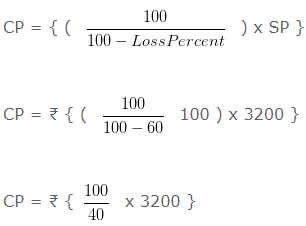CP = ₹ 8000

Hence, CP = ₹ 8000

#### Profit and Loss Questions for Class 7 – Find Cost Price when Selling Price & Gain Percent is given

Question 8

Find the Cost Price of an Article whose Selling Price = ₹ 2550 and Gain% = 25 % ?

Explanation:

Cost Price (CP) – Price at which an Article is purchased.

Selling Price (SP) – Price at which the Article is sold.

Gain – Where the Selling Price is greater than the Cost Price, the difference between the SP and CP is the gain

CP = ?

SP = ₹ 2550

Gain% = 25 %

We know that,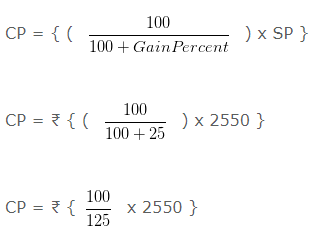CP = ₹ 2040

Hence, CP = ₹ 2040

#### Profit and Loss Questions for Class 7 – Find Selling Price when Cost Price & Loss Percent is given

Question 9

Find the Selling Price of an Article whose Cost Price = ₹ 1500 and Loss % = 20 % ?

Explanation:

Cost price(CP) – Price at which an article is purchased.

Selling price(SP) – Price at which an article is sold.

Loss – Where the Cost Price is greater than the Selling Price, the difference between the SP and CP is the Loss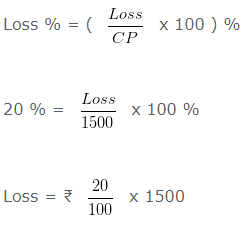= ₹ 300

CP = ₹ 1500

SP = ?

Loss = 300

We know that,

Selling Price (SP) = Cost Price – Loss

= 1500 – 300

= 1200

Hence, SP = ₹ 1200

Alternative Method:

In present case,

CP = ₹ 1500

SP = ?

loss% = 20 %

As we know that,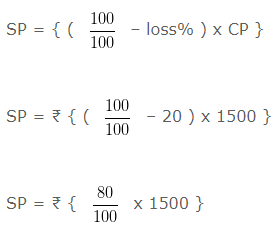SP = ₹ 1200

Hence, SP = ₹ 1200

#### Profit and Loss Questions for Class 7 – Find Selling Price when Cost Price & Gain Percent is given

Question 10

Find the Selling Price of an Article whose Cost Price = ₹ 2400 and Gain % = 50 % ?

Explanation:

Cost Price (CP) – Price at which an Article is purchased.

Selling Price (SP) – Price at which the Article is sold.

Gain – Where the Selling Price is greater than the Cost Price, the difference between the SP and CP is the gain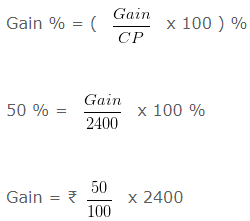Gain = ₹ 1200

CP = ₹ 2400

SP = ?

Gain = ₹ 1200

As we know that,

Selling Price (SP) – = Cost Price + Gain

= ₹ ( 2400 + 1200 )

= ₹ ( 3600 )

Hence, SP = ₹ 3600

1.